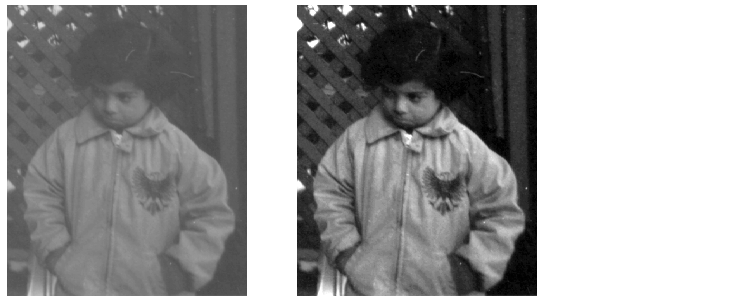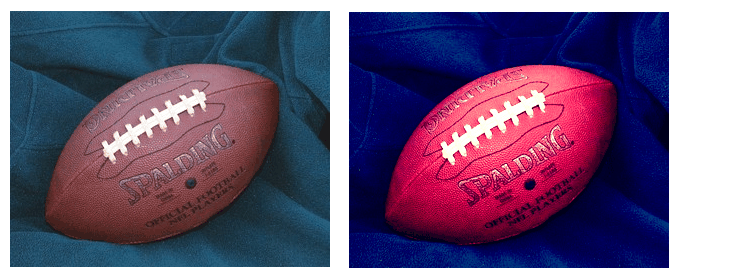Image Processing Toolbox User's GuideAdjust image intensity values or colormap

Syntax

• ```J = imadjust(I)
```

Description

```J = imadjust(I) ``` maps the values in intensity image `I` to new values in `J` such that 1% of data is saturated at low and high intensities of `I`. This increases the contrast of the output image `J`. This syntax is equivalent to `imadjust(I,stretchlim(I))`.

`J = imadjust(I,[low_in; high_in],[low_out; high_out])` maps the values in intensity image `I `to new values in `J` such that values between `low_in` and `high_in` map to values between `low_out` and `high_out`. Values below `low_in` and above `high_in` are clipped; that is, values below `low_in` map to `low_out`, and those above `high_in` map to `high_out`. You can use an empty matrix (`[]`) for [`low_in` `high_in`] or for [`low_out` `high_out`] to specify the default of `[0 1]`.

`J = imadjust(I,[low_in; high_in],[low_out; high_out],gamma)` maps the values in intensity image `I `to new values in `J`, where `gamma` specifies the shape of the curve describing the relationship between the values in `I` and `J`. If `gamma` is less than 1, the mapping is weighted toward higher (brighter) output values. If `gamma` is greater than 1, the mapping is weighted toward lower (darker) output values. If you omit the argument, `gamma` defaults to 1 (linear mapping).

`newmap = imadjust(map,[low_in; high_in],[low_out; high_out],gamma)` transforms the colormap associated with an indexed image. If `low_in`, `high_in`, `low_out`, `high_out`, and `gamma` are scalars, then the same mapping applies to red, green, and blue components. Unique mappings for each color component are possible when

• `low_in` and `high_in` are both 1-by-3 vectors.

`low_out` and `high_out` are both 1-by-3 vectors, or `gamma` is a 1-by-3 vector.

The rescaled colormap `newmap` is the same size as `map`.

`RGB2 = imadjust(RGB1,...)` performs the adjustment on each image plane (red, green, and blue) of the RGB image `RGB1`. As with the colormap adjustment, you can apply unique mappings to each plane.

 Note    If `high_out` `< low_out`, the output image is reversed, as in a photographic negative.

Class Support

For syntax variations that include an input image (rather than a colormap), the input image can be of class `uint8`, `uint16`, `int16`, `single`, or `double`. The output image has the same class as the input image. For syntax variations that include a colormap, the input and output colormaps are of class `double`.

Example

• ```I = imread('pout.tif');
imshow(I), figure, imshow(J)```

Adjust the grayscale image, specifying the contrast limits.

• ```K = imadjust(I,[0.3 0.7],[]);
figure, imshow(K)
```

• ```RGB1 = imread('football.jpg');
RGB2 = imadjust(RGB1,[.2 .3 0; .6 .7 1],[]);
imshow(RGB1), figure, imshow(RGB2)```

`brighten`, `histeq`, `stretchlim`